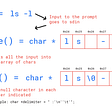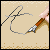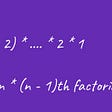# How to Write a C-like sizeof Function in Java?

If you just started learning Java and come from a C background, then you might have noticed a difference between Java and C programming language, like String is an object in Java and not a `NULL` terminated character array. Similarly, there is no `sizeof()` operator in Java. All primitive values have a predefined size, e.g. int is 4 bytes, char is 2 byte, short is 2 byte, long and float is 8 byte, and so on.

But, if you are missing the operator, then why not let's make it a coding task? If you are OK, then your next task is to write a method in Java, which can behave like the `sizeOf()` operator/function in C and returns size in bytes for each numeric primitive type, i.e. all primitive types except Boolean.

Many of you think, why we are not including Boolean? Doesn’t it just need one bit to represent true and false values? Well, I am not including Boolean in this exercise, because the size of Boolean is not strictly defined in Java specification and varies between different JVM (See Java Fundamentals: The Java Language).

Also, for your knowledge, the size of primitives in Java is fixed. It doesn’t depend upon the platform.
So, an int primitive variable will take four bytes in both Windows and Linux, both on 32-bit and 64-bit machines.

Anyway, here is the size and default values of different primitive types in Java for your reference:

Now, it’s up to your creativity to give multiple answers, but we need at least one answer to solve this coding problem. If you guys like this problem, then I might include this on my list of 75 Coding Problems to Crack Any Programming Job interview, drop a note if this is interesting and challenging.

# Java sizeof() Function Example

Here is our complete Java program to implement the `sizeof` operator. It's not exactly size but its purpose is the same. `sizeof` returns how much memory a particular data type takes and this method does exactly that.

`/*** Java Program to print size of primitive data types e.g. byte, int, short, double, float* char, short etc, in a method like C programming language's sizeof** @author Javin Paul*/public class SizeOf{public static void main(String args[]) {System.out.println(" size of byte in Java is (in bytes) :  "+ sizeof(byte.class));System.out.println(" size of short in Java is (in bytes) :"+ sizeof(short.class));System.out.println(" size of char in Java is (in bytes) :"+ sizeof(char.class));System.out.println(" size of int in Java is (in bytes) :"+ sizeof(int.class));System.out.println(" size of long in Java is (in bytes) :"+ sizeof(long.class));System.out.println(" size of float in Java is (in bytes) :"+ sizeof(float.class));System.out.println(" size of double in Java is (in bytes) :"+ sizeof(double.class));}/** Java method to return size of primitive data type based on hard coded values* valid but provided by developer*/public static int sizeof(Class dataType) {if (dataType == null) {throw new NullPointerException();}if (dataType == byte.class || dataType == Byte.class) {return 1;}if (dataType == short.class || dataType == Short.class) {return 2;}if (dataType == char.class || dataType == Character.class) {return 2;}if (dataType == int.class || dataType == Integer.class) {return 4;}if (dataType == long.class || dataType == Long.class) {return 8;}if (dataType == float.class || dataType == Float.class) {return 4;}if (dataType == double.class || dataType == Double.class) {return 8;}return 4; // default for 32-bit memory pointer}/** A perfect way of creating confusing method name, sizeof and sizeOf* this method take advantage of SIZE constant from wrapper class*/public static int sizeOf(Class dataType) {if (dataType == null) {throw new NullPointerException();}if (dataType == byte.class || dataType == Byte.class) {return Byte.SIZE;}if (dataType == short.class || dataType == Short.class) {return Short.SIZE;}if (dataType == char.class || dataType == Character.class) {return Character.SIZE;}if (dataType == int.class || dataType == Integer.class) {return Integer.SIZE;}if (dataType == long.class || dataType == Long.class) {return Long.SIZE;}if (dataType == float.class || dataType == Float.class) {return Float.SIZE;}if (dataType == double.class || dataType == Double.class) {return Double.SIZE;}return 4; // default for 32-bit memory pointer}}Output:size of byte in Java is (in bytes) :  1size of short in Java is (in bytes) :2size of char in Java is (in bytes) :2size of int in Java is (in bytes) :4size of long in Java is (in bytes) :8size of float in Java is (in bytes) :4size of double in Java is (in bytes) :8`

That’s all in this programming exercise of writing a `sizeof` like a method in Java. This is actually tricky because you don't think of taking advantage of the predefined size of Java data types, nor do you think about taking advantage of size constants defined in wrapper classes, like Integer or Double. If you can come across any other way of finding the size of primitive data type then let us know.

--

--

--

## More from Javarevisited

A humble place to learn Java and Programming better.

## How to do Text Summarization in Python?## Tutorial to code a simple shell in C## Linux Desktop Environment Overview and Remote Desktop Configuration — Veesp## 74 days to become a Game Developer. Day 10.## Keeping Track of Database Schema Changes Using Go## Type System## javinpaul

I am Java programmer, blogger, working on Java, J2EE, UNIX, FIX Protocol. I share Java tips on http://javarevisited.blogspot.com and http://java67.com

## Interview questions on Object-Oriented Programming and JAVA## How Recursion Works — step-by-step walkthrough## Time Complexity of Algorithms Explained with Examples